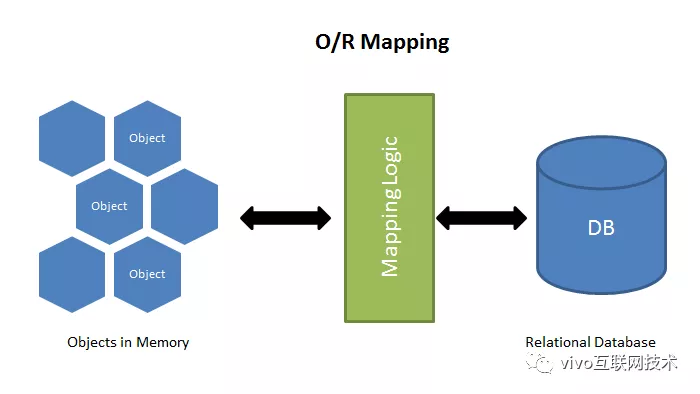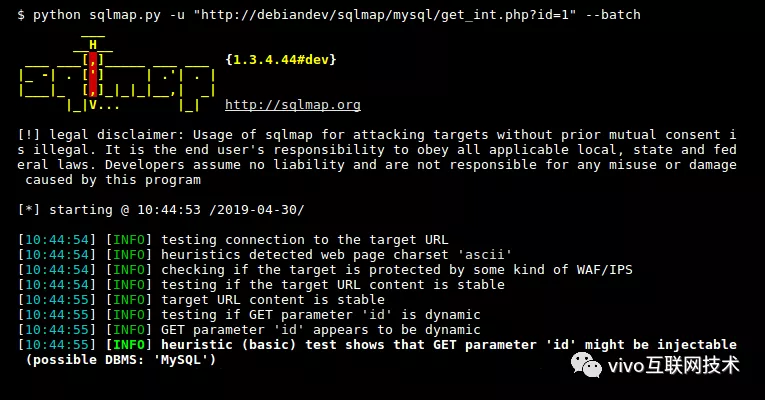—— 题记

# 一、背景

1. 前后端使用 JavaScript 来构建，使得前后端更加融合且高效。前后端代码的复用中部分模块和组件不仅仅可以在前端使用，也可以在后端使用。
2. 减少了大量的沟通成本。围绕着产品的需求设计和迭代，前端工程师在前端和后端的开发上无缝的切换，保证业务的快速落地。
3. 开发者全局开发的视角。让前端的工程师有机会从产品、前端、后端的视角去思考问题和技术的创新。

# 二、Node 数据持久层现状与思考

## 1、纯粹的 MySQL 驱动

Node-MySQL是 Node 连接 MySQL的驱动，使用纯 JavaScript 开发，避免了底层的模块编译。让我们看看如何使用它，首先我们需要安装这个模块。

 1 `npm install mysql`  `# 之前0.9的版本需要这样安装 npm install mysqljs/mysql`1 2 3 4 5 6 7 8 9 10 11 12 13 14 15 16 17 18 19 20 21 22 23 24 25 26 27 28 29 30 31 32 33 34 `var`  `mysql      = require(` `'mysql'` `);` `var`  `connection = mysql.createConnection({` `  ` `host     : ` `'..'` `, ` `// db host` `  ` `user     : ` `'..'` `, ` `// db user` `  ` `password : ` `''` `,   ` `// db password` `  ` `database : ` `'..'`   `// which database` `});`   `connection.connect();`   `connection.query(` `  ` `'SELECT id, name, rank FROM lanaguges'` `,` `  ` `function`  `(error, results, fields) {` `    ` `if`  `(error) ` `throw`  `error;` `    ` `/**` `     ` `* 输出：` `     ` `* [ RowDataPacket {` `     ` `*    id: 1,` `     ` `*    name: "Java",` `     ` `*    rank: 1` `     ` `*  },` `     ` `*  RowDataPacket {` `     ` `*    id: 2,` `     ` `*    name: "C",` `     ` `*    rank: 2` `     ` `*  }` `     ` `*]` `     ` `*` `     ` `*` `     ` `*/` `    ` `console.log(` `'The language rank is: '` `, results);` `});`   `connection.end();`

• 默认使用数据库连接池的方式来提升性能。
• 改进callback的回调函数的风格，迁移到 promise，async/await 更现代化 JavaScript 的异步处理方案。
• 使用更加灵活的事务的处理。
• 针对复杂 SQL 的编写，通过字符串拼接的方式是比较痛苦的，需要更语义化的 SQL 编写能力。

## 2、主流的 ORMORM 把数据库映射成对象：

• 数据库的表（table） => 类（class）
• 记录（row，行数据）=> 对象（object）
• 字段（field）=> 对象的属性（attribute）

Node 在 ORM 的技术方案上，社区有不同的角度的探索，充分体现了社区的多样性，比如目前非常流行的  Sequelize。Sequelize 是一个基于 Promise 的 Node.js ORM， 目前支持 PostgreSQL、MySQL、SQLite 以及 SQL-Server。它具有强大的事务支持、关联关系、预读、延迟加载、读取复制等功能。如上述 MySQL 使用的案例，若使用Sequelize ORM方式来实现，代码如下：

 1 2 3 4 5 6 7 8 9 10 11 12 13 14 15 16 17 18 19 20 21 22 `// 定义ORM的数据与model映射` `const Language = sequelize.define(` `'language'` `, {` `  ` `// 定义id， int型 && 主键` `  ` `id: {` `    ` `type: DataTypes.INTEGER,` `    ` `primaryKey: ` `true` `  ` `},` `  ` `// 定义name, string类型映射数据库varchar` `  ` `name: {` `    ` `type: DataTypes.STRING,` `  ` `},` `  ` `// 定义rank, string类型映射数据库varchar` `  ` `range: {` `    ` `type: DataTypes.INTEGER` `  ` `},` `}, {` `  ` `// 不生成时间戳` `  ` `timestamps: ` `false` `});`   `// 查询所有` `const languages = await Language.findAll()`

## 3、未来之星 TypeORMTypeORM 充分结合 TypeScript，提供更好的开发体验。其目标是始终支持最新的 JavaScript 功能，并提供其他功能来帮助您开发使用数据库的任何类型的应用程序，从带有少量表的小型应用程序到具有多个数据库的大型企业应用程序。

## 4、理性思考

• 对于简单的场景 CRUD 非常快，对于多表和复杂关联查询就会有点力不从心。
• ORM 库不是轻量级工具，需要花很多精力学习和设置。
• 对于复杂的查询，ORM 要么是无法表达，要么是性能不如原生的 SQL。
• ORM 抽象掉了数据库层，开发者无法了解底层的数据库操作，也无法定制一些特殊的 SQL。
• 容易产生N+1查询的问题。

# 三、悟空活动中台在数据持久层的探索

## 1、什么是 MyBatis ？

MyBatis 是一款优秀的持久层框架，它支持自定义 SQL、存储过程以及高级映射。MyBatis 免除了几乎所有的 JDBC 代码以及设置参数和获取结果集的工作。MyBatis 可以通过简单的 XML 或注解来配置和映射原始类型、接口和 Java POJO（Plain Old Java Objects，普通老式 Java 对象）为数据库中的记录。MyBatis 的最棒的设计就是在对象的映射和原生 SQL 强大之间取得了很好的平衡。

SQL 配置

 1 2 3 4 5 6 7 8 `` `` `` `  ` ``

SQL 查询

 1 2 `BlogMapper mapper = session.getMapper(BlogMapper.` `class` `);` `Blog blog = mapper.selectBlog(101);`

• 简单易学。代码实现小而简单。没有任何第三方依赖，易于使用。
• 灵活。Node-Mybatis 不会对应用程序或者数据库的现有设计强加任何影响。借助 ES6 的 string template编写 SQL，灵活直接。
• 解除 SQL 与程序代码的耦合。

• 支持动态 SQL 。避免 SQL 的字符串拼接。
• 防止 SQL 注入。自动对动态参数进行 SQL 防注入。
• 声明式事务机制。借助 decorator 更容易进行事务声明。
• 结合 Typescript 的类型。根据数据的表格结构自动生成数据的类型定义文件，代码提升补齐，提升开发体验。

## 2、Node-MyBatis 解决方案

### （1）SQL-Builder

# 表达式

#：针对动态 SQL中的占位符，我们最经常碰到的场景就是 字符串的占位符，# 后面就是将来动态替换的变量的名称。如：

 1 2 3 4 5 6 7 8 9 10 11 12 13 14 15 16 17 18 19 20 `SELECT` `    ` `id as id,` `    ` `book_name as bookName` `    ` `publish_time as publishTime` `    ` `price as price` `  ` `FROM t_books t` `  ` `WHERE` `    ` `t.id =`  `#data.id AND t.book_name = #data.bookName` `    `  ` ` `-- 该 SQL 通过 Node-MyBatis 底层的 SQL Compile 解析之后，生成的 SQL如下，` ` ` `-- data 参数为: {id: ` `'11236562'` `, bookName: ` `'JavaScript红皮书'`  `}` ` `  ` ` `SELECT` `    ` `id as id,` `    ` `book_name as bookName` `    ` `publish_time as publishTime` `    ` `price as price` `  ` `FROM t_books t` `  ` `WHERE` `    ` `t.id = ` `'11236562'`  `AND t.book_name = ` `'JavaScript红皮书'`

\$ 表达式

\$: 动态数据的占位符，该占位符会在我们的 sql template 编译后将变量的值动态插入 SQL ，如下：

 1 2 3 4 5 6 7 8 9 10 11 12 `SELECT` `  ` `id, name, email` `FROM t_user t` `WHERE t.state=\$data.state AND t.type ` `in`  `(\$data.types)`   `-- 该 SQL 通过 Node-MyBatis 底层的 SQL Compile 解析之后，生成的 SQL如下` `-- data 参数为: {state: 1, types: [1,2,3]}`   `SELECT` `  ` `id, name, email` `FROM t_user t` `WHERE t.state=0 AND t.type ` `in`  `(1,2,3)`

<%%>  代码块

 1 2 3 4 5 6 7 8 9 10 11 12 13 14 15 16 17 18 19 20 21 22 `-- 循环` `SELECT` `    ` `t1.plugin_id as pluginId,` `    ` `t1.en_name  as pluginEnName,` `    ` `t1.version as version,` `    ` `t1.state as state` ` ` `FROM test_npm_list  t1` `    ` `WHERE t1.state = ` `'0'` `  ` `<% ` `for`  `(` `let`  `[name, version] of data.list ) { %>` `     ` `AND t1.en_name =`  `#name AND t1.version=#version` `  ` `<% } %>`   `-- 分支判断` `SELECT` `   ` `id，` `   ` `name,` `   ` `age` `FROM users` `WHERE name like`  `#data.name` `<% ` `if` `(data.age > 10) {%>` `AND age = \$data.age` `<% } %>`

 1 2 3 4 5 6 7 `let`  `template = `` `
` `  ` `<% ` `for` `(` `let`  `i=0; i < data.users.length; i++) { %>` `    ` `
• <%= data.users[i] %>
• ` `  ` `<% } %>` `
` ``;`

 1 2 3 4 5 6 7 `print(` `'
'` `);` `for` `(` `let`  `i = 0; i < data.users.length; i++) {` `  ` `print(` `'
• '` `);` `  ` `print(data.users[i]);` `  ` `print(` `'
• '` `);` `};` `print(` `'
'` `);`

 1 2 3 4 5 6 7 8 9 10 11 12 13 14 15 16 17 18 19 20 21 22 23 24 25 `let`  `evalExpr = /<%=(.+?)%>/g;` `let`  `expr = /<%([\s\S]+?)%>/g;`   `template = template` `  ` `.replace(evalExpr, ` `'`); \n  print( \$1 ); \n  echo(`'` `)` `  ` `.replace(expr, ` `'`); \n \$1 \n  print(`'` `);`   `template = ` `'print(`'`  `+ template + ` `'`);'` `;` `console.log(template);`   `// 输出`   `echo(`` `
` `  ` ``);` `  ` `for` `(` `let`  `i=0; i < data.supplies.length; i++) {` `  ` `echo(`` `    ` `
• `);` `  ` `echo(  data.supplies[i]  );` `  ` `echo(`
• ` `  ` ``);` `  ` `}` `  ` `echo(`` `
` ``);`

 1 2 3 4 5 6 7 8 9 10 11 12 13 14 15 16 17 18 19 20 21 22 23 24 25 `function`  `compile(template){` `  ` `const evalExpr = /<%=(.+?)%>/g;` `  ` `const expr = /<%([\s\S]+?)%>/g;`   `  ` `template = template` `    ` `.replace(evalExpr, ` `'`); \n  print( \$1 ); \n  echo(`'` `)` `    ` `.replace(expr, ` `'`); \n \$1 \n  print(`'` `);`   `  ` `template = ` `'print(`'`  `+ template + ` `'`);'` `;`   `  ` `let`  `script =` `  ` ``(` `function`  `parse(data){` `    ` `let`  `output = ` `""` `;`   `    ` `function`  `print(html){` `      ` `output += html;` `    ` `}`   `    ` `\${ template }`   `    ` `return`  `output;` `  ` `})`;`   `  ` `return`  `script;` `}`

 1 2 3 4 5 6 7 `let`  `parse = eval(compile(template));` `parse({ users: [ ` `"Green"` `, ` `"John"` `, ` `"Lee"`  `] });` `//
` `//
• Green
• ` `//
• John
• ` `//
• Lee
• ` `//
`

 1 2 3 4 5 6 7 8 9 10 11 12 13 14 15 16 17 18 19 20 21 22 23 `sqlCompile(template) {` `    ` `template =` `        ` `'print(`'`  `+` `        ` `template` `            ` `// 解析#动态表达式` `            ` `.replace(/` `#([\w\.]{0,})(\W)/g, '`); \n  print_str( \$1 ); \n  print(`\$2')` `            ` `// 解析\$动态表达式` `            ` `.replace(/\\$([\w\.]{0,})(\W)/g, ` `'`); \n  print( \$1 ); \n  print(`\$2'` `)` `            ` `// 解析<%%>动态语句` `            ` `.replace(/<%([\s\S]+?)%>/g, ` `'`); \n \$1 \n  print(`'` `) +` `        ` `'`);'` `    ` `return`  ``(` `function`  `parse(data,connection){` `      ` `let`  `output = ` `""` `;` `      ` `function`  `print(str){` `        ` `output += str;` `      ` `}` `      ` `function`  `print_str(str){` `       ` `output += ` `"\'"`  `+ str + ` `"\'"` `;` `      ` `}` `      ` `\${template}` `      ` `return`  `output.replace(/[\\r\\n]/g,` `""` `);` `    ` `})`` `  ` `}`

### （2）SQL 防注入

SQL 支持拼接就可能存在 SQL 的注入可能性，Java 中 MyBatis  \$ 动态表达式的使用也是有注入风险的，因为 \$ 可以置换变量不会被包裹字符引号，社区也不建议使用 \$ 符号来拼接 SQL。对于 Node-MyBatis 来说，因为保留了 \$ 的能力，所以需要处理 SQL 注入的风险。参考 MyBatis 的 Node-MyBatis 工具用法也比较简单，示例如下：

 1 2 3 `// data = {name: 1}` ``db.name =`  `#data.name` // => 字符替换，会被转义成  db.name = "1"` ``db.name = \$data.name` ` `// => 完整替换，会被转义成  db.name =  1`

 1 2 3 4 5 6 7 8 9 10 11 `// SQL 模板` ``SELECT * from t_user WHERE username = \$data.name and paasword = \$data.passwd`` `// data 数据为 {username: "'admin' or 1 = 1 --'", passwd: ""}` `// 这样通过 SQL注释构造 形成了SQL的注入` ``SELECT * FROM members WHERE username = 'admin` `' or 1 = 1 -- AND password = '` `'``   `// SQL 模板` ``SELECT * from \$data.table`` `// data 数据为 {table: "user;drop table user"}` `// 这样通过 SQL注释构造 形成了SQL的注入` ``SELECT * from user;drop table user``

escape转义，使用 \$ 的进行传值，模板底层会先走 escape 方法进行转义，我们用一个包含不同的数据类型的数据进行 escape 能力检测，如：

 1 2 3 4 `const arr = escape（[1,` `"a"` `,` `true` `,` `false` `,` `null` `,undefined,` `new`  `Date()]）;`   `// 输出` `( 1,` `'a'` `, ` `true` `, ` `false` `, NULL, NULL, ` `'2019-12-13 16:19:17.947'` `)`

 1 2 3 4 5 6 `if` `(tag === ` `'\$'` `){` `  ` `if` `(/where|select|sleep|benchmark/gi.test(str)){` `    ` `throw`  `new`  `Error(` `'\$ value not allowed include where、select、sleep、benchmark keyword !'` `)` `  ` `}` `  ` `//...` `}`

Connection options - 连接属性

multipleStatements:

• Allow multiple mysql statements per query. Be careful with this, it could increase the scope of SQL injection attacks. (Default: false)

• 每个查询允许多个mysql语句。 请注意这一点，它可能会增加SQL注入攻击的范围。 （默认值：false）

Node-MyBatis 中默认规避了多行执行语句的配置与 \$ 共同使用的场景。

 1 2 3 4 5 6 `if` `(tag === ` `'\$'` `){` `  ` `if` `(` `this` `.pool.config.connectionConfig.multipleStatements){` `    ` `throw`  `new`  `Error(` `'\$ and multipleStatements mode not allowed to be used at the same time !'` `)` `  ` `}` `  ` `//...` `}　`

SQL 注入检测

sqlmap 是一个开源的渗透测试工具，可以用来进行自动化检测，利用 SQL 注入漏洞，获取数据库服务器的权限。它具有功能强大的检测引擎,针对各种不同类型数据库的渗透测试的功能选项，包括获取数据库中存储的数据，访问操作系统文件甚至可以通过外带数据连接的方式执行操作系统命令。sqlmap 支持 MySQL, Oracle, PostgreSQL, Microsoft SQL Server, Microsoft Access, IBM DB2, SQLite, Firebird, Sybase 和 SAP MaxDB 等数据库的各种安全漏洞检测。

sqlmap 支持五种不同的注入模式：

• 基于布尔的盲注 即可以根据返回页面判断条件真假的注入；
• 基于时间的盲注 即不能根据页面返回内容判断任何信息，用条件语句查看时间延迟语句是否执行（即页面返回时间是否增加）来判断；
• 基于报错注入 即页面会返回错误信息，或者把注入的语句的结果直接返回在页面中；
• 联合查询注入 可以使用union的情况下的注入；
•  堆查询注入可以同时执行多条语句的执行时的注入。1 2 3 4 5 `//安装方法` `git clone --depth 1 https:` `//github.com/sqlmapproject/sqlmap.git sqlmap-dev`   `//使用方法` `sqlmap -u ` `'some url'`  `--flush-session --batch --cookie=` `"some cookie"`

• -u 设置想要验证的网站url
• --flush-session 清除过去的历史记录
• --batch 批量验证注入

### （3）声明式事务

 1 2 3 4 5 6 7 8 9 10 11 12 13 14 15 `function`  `log(target, name, descriptor) {` `  ` `console.log(target)` `  ` `console.log(name)` `  ` `console.log(descriptor)` `}`   `@log` `class`  `User {` `  ` `walk() {` `    ` `console.log(` `'I am walking'` `)` `  ` `}` `}`   `const u = ` `new`  `User()` `u.walk()`

 1 2 3 4 5 6 7 8